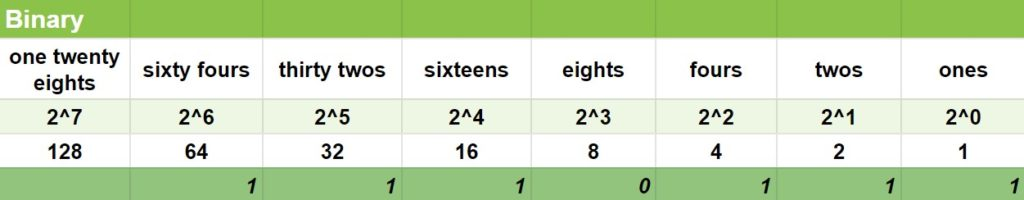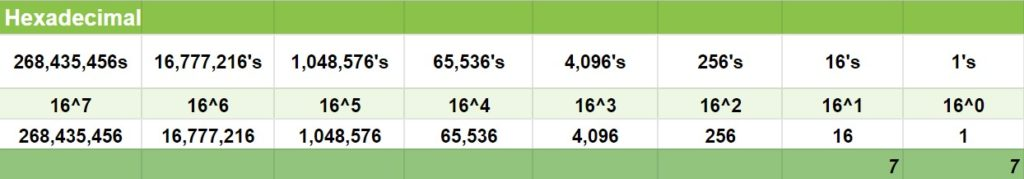Learn more about the generation of computers, switches, binary, hexadecimals, and conventions such as ASCII and UniCode. Know how we measure bits.

Learn more about the generation of computers, switches, binary, hexadecimals, and conventions such as ASCII and UniCode. Know how we measure bits.

## Generation of Computers

### 1. Vacuum Tubes (1904)

John Ambrose Fleming invented the vacuum tubes in 1904. ENIAC (Electronic Numerical Integrator And Computer) was the first computer ever built in history. In the meantime, it had 18,000 tubes! Furthermore, these tubes are to be checked one by one to see if it’s in “on” or “off”.

#### “Debugging”Right: Admiral Grace Hopper. Left: A computer log entry from the Mark II, with a moth taped to the page.

In 1940s, while Admiral Grace Hopper was working on a Mark II computer at Harvard, her associates discovered that a moth was stuck in a relay. Hence, the reason why there was a problem with the computer. So they “debugged” it or removed the bug from the computer in order to fix it.

Today, programmers call a defect, an error, or a problem in a code a “bug.” When they are trying to fix the code that has a “bug“, they are “debugging” it.

### 2. Transistors (1947)

They are way smaller. Unlike the vacuum tubes, they don’t burn out. It can work either as an amplifier or as a switch.

### 3. Integrated Circuits (1959)

They are also known as “chips.” Chips can function as an amplifier, an oscillator, a timer, a microprocessor, and as a memory. In addition, it is a small wafer made of silicon.

### 4. Microprocessors (1971)

Microprocessors were invented by INTEL(Integrated Electronics). Generally, a device that uses a microprocessor is normally capable of many functions, such as word processing, calculation, and communication via Internet or telephone. Moreover, microprocessors act as a device’s “brain“–it transmits, receives and interprets the data needed to operate a device. In short, Microprocessors process data.

### 5. Artificial Intelligence (?)

Today, humans are currently creating, designing, and improving AI. Particularly, it is the creation of intelligent machines that work and react like humans. And it is designed for speech recognition, learning, planning, problem solving, etc. Do you know Siri? She is an AI.

## Switches

For computers, 1 = ON–means energy, electricity, on, power. 0 = OFF–means lack of energy, gone, done, power down. Now these 1s and 0s make up the binary. Bits, which means “binary digits”, are 1s and 0s. Moreover, a byte is made up of eight bits. Hence, eight 1s and 0s. If you would like to know more about the Power Symbol, check this out: Where did the Power Button Symbol come from?

## Numeric System

### Decimal

I think there is no need to explain the decimal system since this is all familiar to us–it’s what we use in our daily lives. But what I wanna let you know too, is that the prefix “deci” means “ten”–that’s why we have numbers 1 up to 10 because “deci” is base-10.

As we move on to the other numeric systems, we’ll understand why people gave them their own prefixes. But before we move on to the next system, I just want you to know that the:

• 10^0 on the table above is just equal to 1;
• 10^1 is equal to 10 (10);
• 10^2 is equal to 100 (10 x 10);
• 10^3 is equal to 1,000 (10 x 10 x 10); and so on.

### Binary

The prefix “bi” in the word “binary” means “two”. That’s why we use this prefix on other things like “bicycle” (because it has two wheels) and “binoculars” (because it has two lenses).

Likewise, in binary, our base number is 2 (two)–0 (OFF) and 1 (ON). Are you familiar with these numbers: 1, 2, 4, 8, 16, 32, 64, 128, 256? Yes, these are digits with the base number 2. That’s why:

• 2^0 is equal to 1;
• 2^1 is equal to 2 (2);
• 2^2 is equal to 4 (2 x 2);
• 2^3 is equal to 8 (2 x 2 x 2);
• 2^4 is equal to 16 (2 x 2 x 2 x 2); and so on.

This is the representation of the decimal number 119 to binary:The decimal 119 is equal to 1110111 in binary.

### How?

So just to understand this:

• If we’re going to put a 1 under the 1 (ones), we’ll have 1;
• If we’ll put 1 under the 2 (twos), we now have 3 (because 1 + 2 = 3);
• And if we put another 1 under the 4 (fours), we now have 7 (because 1 + 2 + 4 = 7).

So how did 119 became 1110111 in binary? Let’s break this to smaller details:

• We put 1 under 1 (ones), so we have 1 (1).
• We put 1 under 2 (twos), so we have 3 (1 + 2).
• We put 1 under 4 (fours), so we have 7 (1 + 2 + 4).
• We put 0 under 8 (eights) because we don’t need that; we still have 7 (1 + 2 + 4 + 0).
• We put 1 under 16 (sixteens), so we have 23 (1 + 2 + 4 + 16).
• We put 1 under 32 (thirty-twos), so we have 55 (1 + 2 + 4 + 16 + 32).
• Lastly, we put 1 under 64 (sixty-fours), so we have 119 (1 + 2 + 4 + 16 + 32 + 64).

“But what if we put 1s (no 0s) on all these placeholders until 128 (one-twenty-eights), like this?

Well what is this statement equal to: 128 + 64 + 32 + 16 + 8 + 4 + 2 + 1 = ?

Yup 255. Now these 0s and 1s are used to encode 256 (from 0 to 255) characters in ASCII. We’ll discuss this later. For now, we’ll first discuss a better, and more efficient way to store data.

### Hexadecimal

Hexadecimals, like all the other numeric systems, has a reason why people gave it this name. We’ve learned that the prefixes:

• “Deci” means “ten”;
• “bi” means “two”;
• “hexa” means “sixteen”.

That’s right. This numeric system is base-16 (0 to 15). And this is how you represent each decimal number to their hexadecimal value:

This is how we’ll represent the decimal number 119 in HEX:These are the values in the hexadecimal system.

Like the first tables shown before this numeric system:

• 16^0 is equal to 1;
• 16^1 is equal to 16 (16);
• 16^2 is equal to 256 (16 x 16);
• 16^3 is equal to 4,096 (16 x 16 x 16); and so on.

Having this knowledge, I challenge you to explain down in the Comments section how 77 (‘HEX 77’ or ‘0x77’) became 119.

## Conventions

### ASCII

ASCII (“American Standard Code for Information Interchange”) is where:

• 0 0 0 0 0 0 0 0 is equal to 0.
• 1 1 1 1 1 1 1 1 is equal to 255.
• 0 0 0 0 1 1 1 1 is equal to 15.

Therefore, if you’re gonna encode and say “Zeddrix T. Fabian” (spaces and period included) in ASCII, it will be: [90, 101, 100, 100, 114, 105, 120, 32, 84, 46, 32, 70, 97, 98, 105, 97, 110]. You should try and encode your name, too. It’s fun!

However, the problem with ASCII is that it only encodes English and maybe a few other similar languages. Remember that a byte can only hold 256 numbers. Turns out, there’s a lot more characters than 256 that is used throughout the world’s languages. The solution?

### Unicode

In comparison, it is like the ASCII table, but it’s huge. You can use 32 bits to encode a Unicode character. Furthermore, 32-bit number means that we can store 4, 294, 267, 295 characters (232), which is enough space for every possible human language and probably a lot of alien ones too. Right now, we use the extra space for important things like poop and smile emojis.

Additionally, it uses a variable bit encoding program where you can choose between 32-bits, 16-bits, and 8-bits encoding.

## Measuring Bits

Usually, we see these things on our devices. And like what is shown on the table above, 1 KiloByte (KB) is equal to 1024 Bytes, not 1000 Bytes. we already have discussed, there are eight bits (0 0 0 0 0 0 0 0) in a Byte. Binary works this way: 1 x 2 = 2; 2 x 2 = 4; 4 x 2 = 8; 8 x 2 = 16; 16 x 2 = 32; 32 x 2 = 64; 64 x 2 = 128; 128 x 2 = 256; 256 x 2 = 512; and finally, 512 x 2 = 1024. (I was able to memorize this because I once played the game 2048).

### Start Learning!

Now that you have a wide understanding of computers, you have the potential to be a good developer. But before we move on to programming and coding (it’s the same thing), kindly move on to the next lesson for some programming reminders.

### Next Lesson: Lesson 2. Important Rules and Reminders for All Programmers

This article was written by Zeddrix Fabian

### About Zeddrix FabianZeddrix Fabian became one of Jehovah’s Witnesses on February 21, 2016, and started regular pioneering last March 2020. His goal is to be able to volunteer at Bethel. After going to both private and public school for 10 years (Grade 1 to 8 in private; Grade 9 and 10 in public school), their family tried out homeschooling when he leveled up to Grade 11 last 2019. (Continue reading on the ABOUT ZEDD menu.)

## 6 thoughts on “Lesson 1. Computers and Its Generation”

1.Remy Garcia says:

In my age I never know what some of your words explain. But I realize you’re learning a lot!

1.Zeddrix Fabian says:

Thank you Ate Remy for giving your time to take a look at my blog!